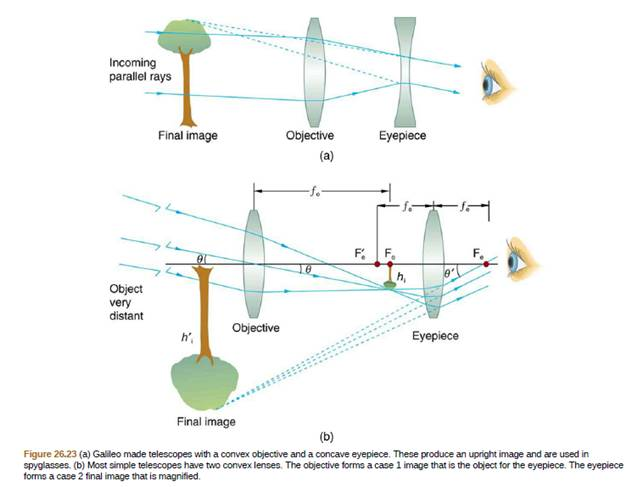Chapter 26, Problem 38PE

Chapter
Section
Textbook Problem

Construct Your Own ProblemConsider a telescope of the type used by Galileo, having a convex objective and a concave eyepiece as Illustrated in Figure 26.23(a). Construct a problem in which you calculate the location and size of the image produced. Among the things to be considered are the focal lengths of the lenses and their relative placements as well as the size and location of the object. Verity that the angular magnification is greater than one. That is, the angle subtended at the eye by the image is greater than the angle subtended by the object.To determine

Construct a problem and show that the angular magnification is greater than one for the problem.

Explanation

We can construct a problem like below: -

The figure of a movie like Star Wars is 10.0 cm tall, is placed 25.0 cm in front of a concave mirror with a focal length of 10.0 cm. Where is the image? How tall is the image? What are the characteristics of the image?

The location of the image can be found from the mirror equation:

1d0+1di=1f1di=1f1d0

which can be rearranged to:

1di=110125=2510250=15250=0.06di=16

Still sussing out bartleby?

Check out a sample textbook solution.

See a sample solution

The Solution to Your Study Problems

Bartleby provides explanations to thousands of textbook problems written by our experts, many with advanced degrees!

Get Started

Find more solutions based on key concepts